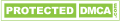# Vortex Flow meter Measuring Principle

The flow measurement of vortex flow transducer is according to the principle of Karman Vortices. When a bluff body is placed vertically in pipe, as the fluid flows around the bluff body, regular vortices columns will be generated alternately on each side of the bluff body (shown as Fig.1), these eddies known as “Karman Vortices”. The resistance part shedding the vortices is named “bluff body”. The frequency of the vortices shedding is related to the velocity of the fluid. Expressed by formula as below: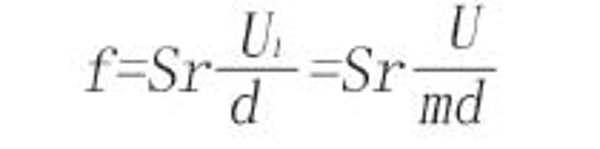Thereinto: f----frequency of Karman Vortices shedding

Sr----Strouhal number

U---- average velocity in the pipe

d----width of bluff body inflow face

m----the ratio of the flow-area sum on both side and measuring pipe section area

U1----average velocity on two sides of bluff body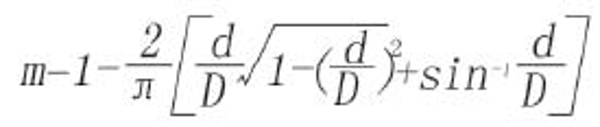Thereinto: D----inside diameter of pipe

d----width of bluff body inflow face (unit: mm)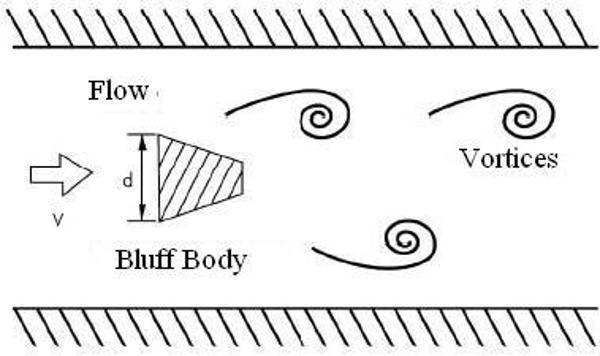Fig.1

Strouhal number is related with the shape of bluff body and Reynolds number (shown as chart 2). From the chart, Sr is constant basically when the range of Reynolds number is ~ .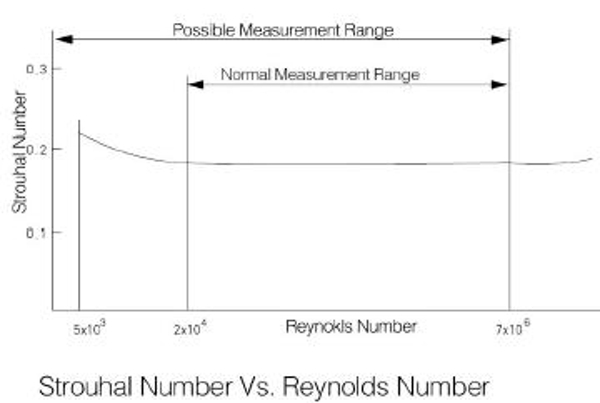Chart 2

Instantaneous volumetric flow qformula as below: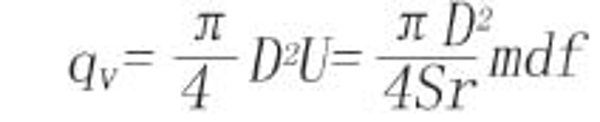Meter factor K formula as below: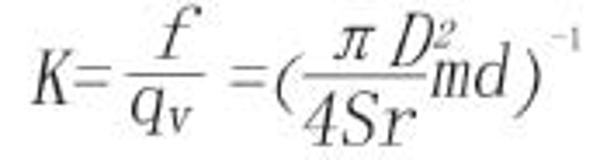Thereinto: q----volumetric flowrate through flow transducer (unit: m3/s)

f----vortices frequency (unit: Hz)

k----meter factor of vortex flow transducer (unit: m)

U----average velocity in the pipe (unit: m/s)

Chart 2 shows that the volumetric flowrate of vortex flow meter is proportional to the vortices frequency. Meter factor K is only related with geometry parameter of bluff body, not related with physical character of fluid and ingredients.

The common formula of vortex flowmeter in the project as below:Thereinto: f----releasing frequency of Karman Vortices (unit: Hz)

q----volumetric flowrate (unit: m3/h)

The introduction of Strouhal number Sr :

Strouhal number is a very important factor in the Vortex Flow Transducer. In the certain range of Reynolds number, Strouhal number approaches the constant. In the range of straight region of Sr≈0.17 in curve (shown as chart 2), frequency of vortices shedding is proportional to the velocity, so as long as the frequency (f) be detected, the velocity (v) will be obtained, and volumetric flowrate will be got according to v. When the fluid flows in pipeline and the Reynolds number is between ~ , Strouhal number is seen as the constant, which can guarantee the accuracy of measuring range. If out of the range of Reynolds number above, Sr will increase as Reynolds number decreases or increases, the nonlinearity will happen. When Reynolds number decrease from to , although vortices shed, the measuring accuracy will be reduced , because of nonlinearity. So Reynolds number ~ will be referred as the measuring range of Vortex flow transducer.

Related Articles